Next: Summarizing Up: Adding Computation Domains Previous: Data Structures in General

Subsections

# Putting Everything Together

We will now develop a couple of small examples in which we will mix constraint handling and the use of data structures. This is a combination available in all CLP systems, and increases the constraints part of the language with the possibility of structuring data; at the same time, it allows the setting up of constraints among components of data structures.

## Systems of Linear Equations

Our first example will use lists to construct a very simple interface to the constraint solver. In fact, this will not add anything to the capabilities of the solver: linear systems can be solved just by writing them in the prompt, like in

?- 3 * X + Y = 5, X + 8 * Y = 3.
Y = 4/23, X = 37/23


But in a program we will probably want to manipulate the coefficients and the solutions as a whole. Data structures will allow us to define systems of equations in a simple way, and manage them as a data structure which can be handled, transformed, and accessed at will.

We will first code a procedure for making dot product of vectors, mathematically defined as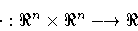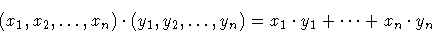The first problem is how to represent vectors. A customary and easy representation uses lists, where each element is one of the coordinates of the vector. Therefore we can write our code as follows:

prod([], [], 0).
prod([X|Xs], [Y|Ys], X * Y + Rest):-
prod(Xs, Ys, Rest).


We are adopting the convention (very convenient, for our case) that multiplying two vectors of zero dimensions yields zero as result. The product of two vectors is then worked out by multiplying elements pairwise and adding this to the result of multiplying the elements in the rest of the vector. The code behaves as expected:

?- prod([2, 3], [4, 5], K).
K = 23

?- prod([2, 3], [5, X2], 22).
X2 = 4


Note that it multiplies, but it also finds values of coordinates which satisfy a multiplication. This can be directly used to solve equation systems:

?- prod([3,1], [X,Y], 5),
prod([1,8], [X,Y], 3).
Y = 4/23, X = 37/23.


but it is not yet what is needed: processing each equation is done with a separate call, and free coefficients are not grouped together. Another predicate, which takes a matrix of factors, a vector of variables, and a vector of free coefficients will do the job:

system(_Vars, [], []).
system(Vars, [Co|Coefs], [Ind|Indeps]):-
prod(Vars, Co, Ind),
system(Vars, Coefs, Indeps).


Matrices are expressed as lists of vectors, and vectors as lists of numbers. Calls to the predicate can be now made, and all the needed data is packed in separate data structures:

?- system([X,Y], [[3,1],[1,8]], [5,3]).
Y = 4/23, X = 37/23.


## Analog RLC circuits

We will develop a simple program which receives a data structure (which we will write down directly, but which could be constructed from reading a description file) and information about the voltage, current, and frequency. The program will try to find out values for the variables so that all these parameters match together. We will suppose the circuit is in steady state, so that transients have not to be considered. We will also consider that elements are connected either in series or in parallel, so that Ohm laws will suffice--no Kirchoff analysis is needed. If you do not know what all this means, do not be intimidated: it is pretty easy.

The entry point will be the predicate circuit(C, V, I, W), which states that across the network C (this is where the data structure goes), the voltage is V, the current is I and the frequency is W. Voltage and current are complex numbers: this is needed because we will be dealing with inductors and capacitors, which react differently with different frequencies, and the frequency will be kept in the imaginary part.

We will model complex numbers using the c/2 structure; the number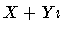will be represented as c(X, Y), and we will implement the addition and multiplication (which, since we are using constraints, implicitly perform subtraction and division) as

c_add(c(Re1,Im1), c(Re2,Im2), R):-
R = c(Re1+Re2,Im1+Im2).
c_mult(c(Re1,Im1),c(Re2,Im2),c(Re3,Im3)):-
Re3 = Re1 * Re2 - Im1 * Im2,
Im3 = Re1 * Im2 + Re2 * Im1.


We will now have a look at composing the parts of the circuit. On one hand we have individual components which behave according to certain laws relating voltage, current, and frequency; these components can be connected among them to build larger units, and these units can again be connected to make bigger circuits. We will use functors for each simple components, which will give their characteristics, as well as for grouping these elements together.

The Ohm laws state that two circuits in series have the same current running through them, they have the same frequency, but the voltage in the endpoints is the sum of the voltages at the endpoints of both circuits:

circuit(series(N1, N2), V, I, W):-
circuit(N1, V1, I, W),
circuit(N2, V2, I, W).


The situation for circuits in parallel is the complementary: the voltage at the endpoints is the same in both, and the same as in their connection in parallel, but the total current of the circuit is divided between them. The frequency is the same in both sub circuits:

circuit(parallel(N1, N2), V, I, W):-
circuit(N1, V, I1, W),
circuit(N2, V, I2, W).


We now have to find out how simpler components react to the conditions they are subject to. We will consider resistors, capacitors, and inductors:

• A resistor obeys the Ohm law V = I * (R + 0i), where V is the voltage, I is the current, R is its resistance, and the frequency is not important. We will model a resistor with the structure resistor(R):
circuit(resistor(R), V, I, W):-
c_mult(I, c(R, 0), V).


• Inductors follow the law V = I * (0 + WLi), where W is the frequency and L the inductor's inductance. Similarly to resistors, they are modeled as
circuit(inductor(L), V, I, W):-
c_mult(I, c(0, W * L), V).


• And finally, capacitors meet the equation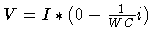, where C is the capacitance. The corresponding clause is
circuit(capacitor(C), V, I, W):-
c_mult(I, c(0, -1 / (W * C)), V).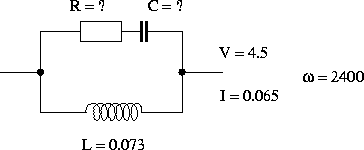Figure 3.5 shows a circuit, where ?'' denotes unknown values. A query which models the circuit in a data structure and automatically finds out the unknown values is as follows:

?- circuit(parallel(inductor(0.073), series(capacitor(C), resistor(R))),
c(4.5, 0), c(0.65, 0), 2400).
R = 24939720/3608029, C = 3608029/2365200000.


This solution is possible, of course, because the execution converts the traversal of the data structure into a set of equations which are linear and which can be solved directly. If the equation system were not linear, a more sophisticated solving method (for example, a nonlinear solver, probably with the help of some search method to isolate a solution) would be needed. The equations generated depend on the description of the circuit, which is input data.Next: Summarizing Up: Adding Computation Domains Previous: Data Structures in General
MCL
1998-12-03